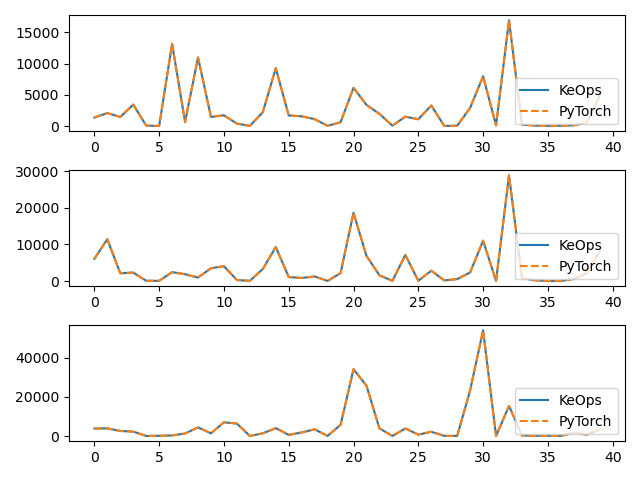# Sum reduction

Let’s compute the (3000,3) tensor $$c$$ whose entries $$c_i^u$$ are given by:

$c_i^u = \sum_j (p-a_j)^2 \exp(x_i^u+y_j^u)$

where

• $$x$$ is a (3000,3) tensor, with entries $$x_i^u$$.

• $$y$$ is a (5000,3) tensor, with entries $$y_j^u$$.

• $$a$$ is a (5000,1) tensor, with entries $$a_j$$.

• $$p$$ is a scalar, encoded as a vector of size (1,).

## Setup

Standard imports:

import time

import matplotlib.pyplot as plt
import torch

from pykeops.torch import Genred


Declare random inputs:

M = 3000
N = 5000

# Choose the storage place for our data : CPU (host) or GPU (device) memory.
device = torch.device("cuda" if torch.cuda.is_available() else "cpu")

dtype = "float32"  # Could be 'float32' or 'float64'
torchtype = torch.float32 if dtype == "float32" else torch.float64

x = torch.randn(M, 3, dtype=torchtype, device=device)
y = torch.randn(N, 3, dtype=torchtype, device=device, requires_grad=True)
a = torch.randn(N, 1, dtype=torchtype, device=device)
p = torch.randn(1, dtype=torchtype, device=device)


## Define a custom formula

formula = "Square(p-a)*Exp(x+y)"
variables = [
"x = Vi(3)",  # First arg   : i-variable, of size 3
"y = Vj(3)",  # Second arg  : j-variable, of size 3
"a = Vj(1)",  # Third arg   : j-variable, of size 1 (scalar)
"p = Pm(1)",
]  # Fourth  arg : Parameter,  of size 1 (scalar)


Our sum reduction is performed over the index $$j$$, i.e. on the axis 1 of the kernel matrix. The output c is an $$x$$-variable indexed by $$i$$.

my_routine = Genred(formula, variables, reduction_op="Sum", axis=1)
c = my_routine(x, y, a, p)


Now, let’s compute the gradient of $$c$$ with respect to $$y$$. Since $$c$$ is not scalar valued, its “gradient” $$\partial c$$ should be understood as the adjoint of the differential operator, i.e. as the linear operator that:

• takes as input a new tensor $$e$$ with the shape of $$c$$

• outputs a tensor $$g$$ with the shape of $$y$$

such that for all variation $$\delta y$$ of $$y$$ we have:

$\langle \text{d} c . \delta y , e \rangle = \langle g , \delta y \rangle = \langle \delta y , \partial c . e \rangle$

Backpropagation is all about computing the tensor $$g=\partial c . e$$ efficiently, for arbitrary values of $$e$$:

# Declare a new tensor of shape (M,3) used as the input of the gradient operator.
# It can be understood as a "gradient with respect to the output c"
# and is thus called "grad_output" in the documentation of PyTorch.
e = torch.rand_like(c)

start = time.time()

# PyTorch remark : grad(c, y, e) alone outputs a length 1 tuple, hence the need for .
g = grad(c, y, e)  # g = [∂_y c].e

print(
"Time to compute gradient of convolution operation with KeOps: ",
round(time.time() - start, 5),
"s",
)


Out:

Time to compute gradient of convolution operation with KeOps:  0.00092 s


The equivalent code with a “vanilla” pytorch implementation

g_torch = (
(
(p - a.transpose(0, 1))[:, None] ** 2
* torch.exp(x.transpose(0, 1)[:, :, None] + y.transpose(0, 1)[:, None, :])
* e.transpose(0, 1)[:, :, None]
)
.sum(dim=1)
.transpose(0, 1)
)

# Plot the results next to each other:
for i in range(3):
plt.subplot(3, 1, i + 1)
plt.plot(g.detach().cpu().numpy()[:40, i], "-", label="KeOps")
plt.plot(g_torch.detach().cpu().numpy()[:40, i], "--", label="PyTorch")
plt.legend(loc="lower right")
plt.tight_layout()
plt.show()Total running time of the script: ( 0 minutes 0.125 seconds)

Gallery generated by Sphinx-Gallery